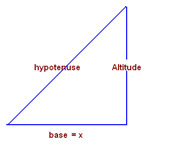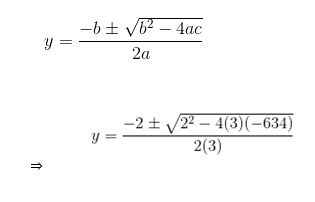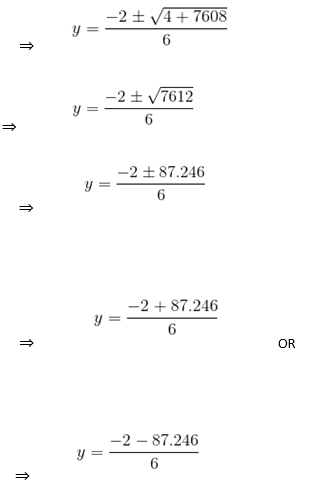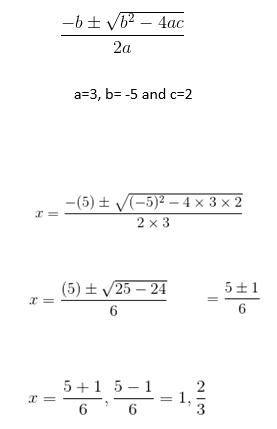# CBSE Class 10 Maths Chapter 4 Quadratic Equations Objective Questions

CBSE Class 10 Maths Chapter 4 Quadratic Equations Objective Questions help students learn how to find out the unknown values of x. Chapter 4 – Quadratic Equations of Class 10 Maths deals with explanations of how to find the value of x from a given equation when x is of unknown value, and a, b, and c are of known value. The theory found in the concept discussed is that an equation to be quadratic “a” should not be equal to 0. However, the equation is of the form ax2 + bx + c = 0. Also, the values of a, b, and c are always real numbers. Now, as per the latest exam pattern, more MCQs are expected to appear in the board exam question paper. So, we have compiled some 20 objective questions from Chapter 4 in this CBSE Class 10 Maths Chapter 4 Quadratic Equations Objective Questions, which the students can solve for practice.

### List of Sub-Topics Covered in Chapter 4

The MCQs for CBSE Class 10 Maths Chapter 4 Quadratic Equations are prepared from the board examination perspective. We have also given the list of topics which are covered in these CBSE Class 10 Maths objective type of questions.

4.1 Introduction to Quadratic Equations (5 MCQs from This Topic)

4.2 Solving QE by Factorisation (5 MCQs from The Topic)

4.3 Solving QE by Completing the Square (5 MCQs from This Topic)

4.4 Solving QE Using the Quadratic Formula (5 MCQs from This Topic)

1. What is the degree of a quadratic equation?
1. 0
2. 2
3. 3
4. 1

Solution: The standard form of the quadratic equation is ax2 + bx + c = 0, a ≠ 0. So the degree of a quadratic equation is 2.

1. Find the sum of the roots of the equation x2–8x+2 = 0
1. 8
2. -8
3. 2
4. -6

Solution: For general quadratic equation ax2+bx+c = 0.

The sum of the roots = −b/a

For x2–8x+2 = 0

The sum of the roots = − (−8/1) =8

The sum of the roots of the equation is 8

1. Which of the following is not a quadratic equation?
1. x(2x + 3) = x2+ 1
2. x(x + 1) + 8 = (x + 2) (x – 2)
3. (x+2)3 = x3-4
4. (x–2)2+ 1 = 2x -3

Answer: (B) x(x + 1) + 8 = (x + 2) (x – 2)

Solution:

(a) x(2x + 3) = x2 + 1

2x2 + 3x = x2+1

x2 +3x -1 = 0

(b) x(x + 1) + 8 = (x + 2) (x – 2)

x2 + x + 8 = x2 – 4

x+12 = 0

This is not a quadratic equation.

(c)  (x+2)3 = x3 – 4

x+ 6x2 + 12x + 8 = x3 – 4

6x+ 12x + 12 = 0

(d) (x−2)2+ 1 = (2x – 3)

x2 – 4x + 4 + 1 = 2x – 3

x2 – 4x + 4 + 1 – 2x + 3 = 0

x2 – 6x + 8 = 0

1. If the sum of the roots of a quadratic equation is 5 and the product of the roots is also 5, then the equation is
1. x2+10x+5 = 0
2. x2–5x+5 = 0
3. x2+5x–5 = 0
4. x2–5x+10 = 0

Solution:  For a quadratic equation ax2+bx+c = 0,

sum of roots = −ba

product of roots = ca.

sum of roots =5 = −ba

product of roots = 5 = ca,

Thus, the quadratic equation is x2−5x+5=0

1. A rectangular field has an area of 3 sq. units. The length is one more than twice the breadth ‘x’. Which of the following will be an equation to represent this?
1. x2 – 2x+6 = 0
2. x2 – 2x+3 = 0
3. 2x2+x−3 = 0
4. 2x2+x−6 = 0

Solution: Area of rectangle = length×breadth

Given, length = (2×breadth + 1)

Let the breadth of the field be x.

Length of the field = 2x+1

Area of the rectangular field = x (2x+1) = 3

2x2+x=3

2x2+x−3=0

### Solving QE by Factorisation

1. The roots of the quadratic equation x2+5x-14 = 0 are
1. 2, 7
2. -2, 7
3. -2, -7
4. 2, -7

Solutions: x2+5x-14 = 0

We need to split the coefficient of x such that the sum of the factors is 5, and their product is -14.

So, we will find the coefficient as 7 and -2.

The sum of 7 and -2 is 5, and the product is -14.

So now re-write the equation

x2+7x-2x-14=0

Taking common terms out

x(x+7)-2(x+7) =0

Again taking out the common terms

(x-2)(x+7)=0

Now, equate the factors to zero to find the roots.

So the roots of the equation are 2,-7

1. Factorise x2 +5x+6 = 0
1. (x-1) (x-3)
2. (x+1) (x+3)
3. (x-2) (x-3)
4. (x+2) (x+3)

Solution: Comparing x2 +5x+6 =0 to ax2 + bx+ c = 0, we have a = 1, b = 5 and c = 6

Now, we need to find two numbers whose product is 6 and whose sum is 5

Pairs of numbers whose product is 6

1, 6

-1,-6

2, 3

-2,-3

Of these pairs, the pair that gives the sum 5 is the third pair

Identifying the pair, we rewrite the given quadratic equation as

x2 +5x+6= x2 +2x+3x+6 =  x(x+2) +3(x+2) = (x+2) (x+3).

1. The altitude of a right triangle is 7 cm less than its base. If the hypotenuse is 13 cm, find the other two sides (in cm).
1. 12, 5
2. 7,2
3. 5,3
4. 2,5

Solutions:Let the base =   x cm

Given that the altitude of a right triangle is 7 cm less than its base

Altitude is =   x – 7 cm

Given that hypotenuse = 13cm

Applying Pythagoras’ theorem,

base2+ altitude2 = hypotenuse2

Substituting the values, we get

⇒   x2 + (x−7)2 = 132

⇒    x2 + x2 + 49 – 14x = 169

⇒   2x2 – 14x + 49 – 169 = 0

⇒   2x2 – 14x – 120 = 0

Dividing with 2 on both sides, the above equation simplifies to

⇒   x2 – 7  x  – 60 = 0

⇒   x2 – 12  x + 5  x – 60 = 0

⇒  x (x – 12) + 5 (  x – 12) = 0

⇒  (x – 12) (x + 5) = 0

⇒ x – 12 = 0 or   x + 5 = 0

⇒  x = 12 or   x = –5

Length cannot be negative, so x cannot be equal to – 5

base  x = 12 cm; altitude = 12 – 7 = 5 cm

1. If a train travelled 5 km/hr faster, it would take one hour less to travel 210 km. The speed of the train is:
1. 60 km/hr
2. 70 km/hr
3. 35 km/hr
4. 30 km/hr

Solution: Let the speed of the train be x km/hr.

Distance travelled = 210 km

The time taken to travel 210 km = 210/x hours

When the speed is increased by 5 km/h, the new speed is (x+5)

Time taken to travel 210 km with the new speed is 210 / (x+5) hours

According to the question,

210/x−210/(x+5)=1

⇒210(x+5)−210x=x(x+5)

⇒210x+1050−210x=x2+5x

⇒x2+5x−1050=0

⇒(x+35)(x−30)=0

⇒x= −35, 30

The speed cannot be negative. Thus, the speed of the train is 30 km/hr

1. If the solutions of the equation  x2+3x−18 = 0 are -6, 3, then the roots of equation 2(x2+3x−18) = 0 are
1. 3, 3
2. -6, 3
3. -12, 6
4. -6, 6

Solution: The roots of a quadratic equation do not change when it is multiplied by a constant non-zero real number. So when the equation x2+3x−18 = 0 is multiplied by 2, the roots still remain the same, i.e. -6, 3.

### Solving QE by Completing the Square

1. The square of (5x + 1) is equal to 16. What is x?
1. x = 4, ¼
2. x = -1, 3/5
3. x = 1, 3/2
4. x= -1, 4/5

Answer: (B) x = -1, 3/5

Solution: Converting statement into an equation,

⇒ (5x+1)2 = 16 (Applying (a+b)2 formula)

⇒5x + 1 = ± 4 (Taking square root on both sides)

⇒5x = -5, 3

⇒x = −1, 3/5

1. Using the method of completion of squares, find one of the roots of the equation 2x2−7x+3 = 0. Also, find the equation obtained after the completion of the square.
1. 6, (x−7/4)2−25/16 = 0
2. 3, (x−7/4)2−25/16 = 0
3. 3, (x−7/2)2−25/16 = 0
4. 13, (x−7/2)2−25/16 = 0

Answer: (B) 3, (x−7/4)2−25/16 = 0

Solution: 2x2−7x+3 = 0

Dividing by the coefficient of x2, we get

x2−7/2x+3/2=0; a=1, b=7/2, c=3/2

Adding and subtracting the square of b/2 = 7/4 (half of the coefficient of x)

We get, [x2−2 (7/4) x+ (7/4)2] − (7/4)2+3/2 = 0

The equation after completing the square is:

(x−7/4)2−25/16=0

Taking square root, (x−7/4) = (±5/4)

Taking positive sign 5/4, x = 3

Taking negative sign −5/4, x = 1/2

1. Find the roots of the equation 5x2–6x–2 = 0 by the method of completing the square.
1. x = 3
2. x = (5± √19)/3
3. x = (3± √19)/5
4. x = 5

Answer: (C) x = (3± √19)/5

Solution: Multiplying the equation throughout by 5, we get 25x2–30x–10 = 0

This is the same as:

(5x) 2–[2× (5x) ×3] +32–32–10 = 0

⇒ (5x–3)2–9–10 = 0

⇒(5x–3)2–19 = 0

⇒(5x–3)2 = 19

⇒5x−3 = ± √19

⇒x = (3± √19)/5

1. There is a natural number x. Write down the expression for the product of x and its next natural number.
1. 2x2+1
2. x2– x
3. x2+ x
4. ( x + 1)( x+2)

Solution: If a natural number is x, the next natural number is greater than x by 1, and hence x+1; for example, for 3, the next natural number is 4.  The product of the 2 numbers is x(x+1) = x2+ x.

1. What number should be added to x2+6x to make it a perfect square?
1. 36
2. 18
3. 9
4. 72

Solution: The identity (a+b) 2= (a2+2ab+b2) represents a perfect square.

If we observe carefully, we can see that x2+6x can be written as (a2+2ab+b2) by adding a constant.

x2+2(x) (3) + constant.

To make x2+6x a perfect square, divide the coefficient of x by 2 and then add the square of the result to make this a perfect square.

Hence, 6/2 = 3 and 32 = 9

We should add 9 to make x2+6x a perfect square.

### Solving QE Using the Quadratic Formula

1. The equation x2+4x+c=0 has real roots, then
1. C ≥ 6
2. C ≤ 8
3. C ≤ 4
4. C ≥ 4

Solution: Step 1: For, x2+4x+c = 0, value of discriminant D = 42–4c=16−4c

Step 2: The roots of the quadratic equation are real only when D ≥ 0

16–4c ≥ 0

Step 3:  c ≤ 4

1. Find the discriminant of the quadratic equation 3x2–5x+2 = 0 and hence, find the nature of the roots.
1. –1, no real roots
2. 1, two equal roots
3. –1, two distinct real roots
4. 1, two distinct real roots

Answer: (D) 1, two distinct real roots

Solution: D = b2–4ac = (–5)2–4×3×2=1>0

D = 1>0⇒ Two distinct real roots.

1. Taylor purchased a rectangular plot of area 634 m2. The length of the plot is 2 m more than thrice its breadth. Find the length and breadth (approximate values).
1. 34.6 m & 11.20 m
2. 44.6 m & 14.20 m
3. 32 m & 16 m
4. 88 m & 24 m

Answer: (B) 44.6 m & 14.20 m

Solution: Let x and y be the length and breadth of the rectangle, respectively.

Given, x=2+3y

Area of the rectangle=length × breadth

=xy

⇒634= (2+3y) y

⇒634=2y+3y2

So, 3y2+2y−634=0

The roots of the above quadratic equation will be⇒ y = 14.20  or

y = -14.87

Considering the positive value for breadth, we have y = 14.20.

Using x=2+3y, we have

x=2+3(14.20) = 44.6

Now, we have

x=44.6 and y = 14.20 (approximately).

1. If the equation x2+2(k+2) x+9k=0 has equal roots, then values of k are __________.
1. 1, 4
2. -1, 5
3. -1, -4
4. 1, -5

Solution: Step 1: For, x2+2(k+2) x+9k = 0, value of discriminant D = [2(k+2)] 2–4(9k) =4(K2+4−5k)

Step 2: The roots of the quadratic equation are real and equal only when D = 0

k2+4−5k = 0

⇒k2−5k+4 = 0

⇒k2−k−4k+4 = 0

⇒k(k−1)−4(k−1) = 0

⇒(k−1)(k−4) = 0

Step 3: k = 4 or 1

1. Find the roots of the 3x2 – 5x + 2 = 0 quadratic equation using the quadratic formula.
1. (7±1)/6
2. (4±1)/6
3. (5±2)/6
4. (5±1)/6

Solution: Quadratic equation of the form ax2 + bx + c = 0

The roots of the above quadratic equation will be## CBSE Class 10 Maths Chapter 4 Extra MCQs

1. Which are the two consecutive odd positive integers, the sum of whose square is 290?
(a) 13, 15
(b) 7, 9
(c) 11, 13
(d) 5, 7

2. The 7th and 13th terms of an A.P. are 34 and 64, respectively. Then, what is the 18th term?
(a) 90
(b) 89
(c) 87
(d) 88

Stay tuned for further updates on CBSE Exam. Download BYJU’S – The Learning App and subscribe to the YouTube channel to access interactive Maths and Science videos.